Zum Inhalt springen

## Introduction

TikZ is probably the most complex and powerful tool to create graphic elements in LaTeX. Starting with a simple example, this article introduces some basic concepts: drawing lines, dots, curves, circles, rectangles etc.

Firstly, load the tikz package by including the line \usepackage{tikz} in the preamble of your document, then draw a graphic using the tikzpicture environment.

\documentclass{article}
\usepackage{tikz}
\begin{document}
\begin{tikzpicture}
\draw[gray, thick] (-1,2) -- (2,-4);
\draw[gray, thick] (-1,-1) -- (2,2);
\filldraw[black] (0,0) circle (2pt) node[anchor=west]{Intersection point};
\end{tikzpicture}
\end{document}


This example produces the following output: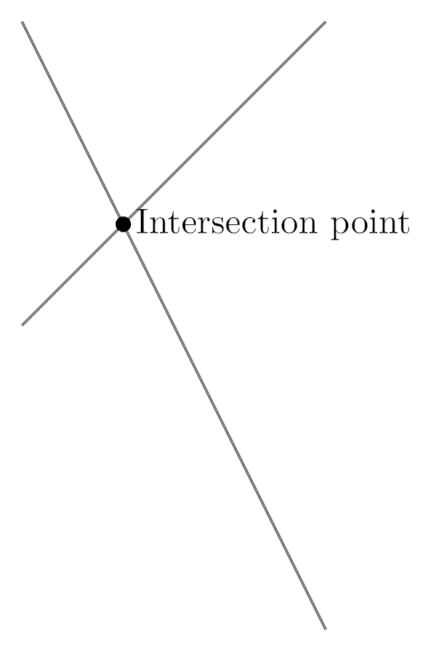In this example two lines and one point are drawn. To add a line the command \draw[gray, thick] defines a graphic element whose colour is gray and with a thick stroke. The line is actually defined by it's two endpoints, (-1,2) and (2,-4), joined by --.

The point is actually a circle drawn by \filldraw[black], this command will not only draw the circle but fill it using black. In this command the centre point (0,0) and the radius (2pt) are declared. Next to the point is a node, which is actually a box containing the text intersection point, and anchored at the west of the point.

It's important to notice the semicolon ; used at the end of each draw command.

Note: The tikzfigure environment can be enclosed inside a figure or similar environment. See the Inserting Images article for more information on this topic.

## Basic elements: points, lines and paths

In this section we provide some examples showing how to create some basic graphic elements which can be combined to create more elaborate figures.

\documentclass{article}
\usepackage{tikz}
\begin{document}
\begin{tikzpicture}

\draw (-2,0) -- (2,0);
\filldraw [gray] (0,0) circle (2pt);
\draw (-2,-2) .. controls (0,0) .. (2,-2);
\draw (-2,2) .. controls (-1,0) and (1,0) .. (2,2);

\end{tikzpicture}
\end{document}


This example produces the following output: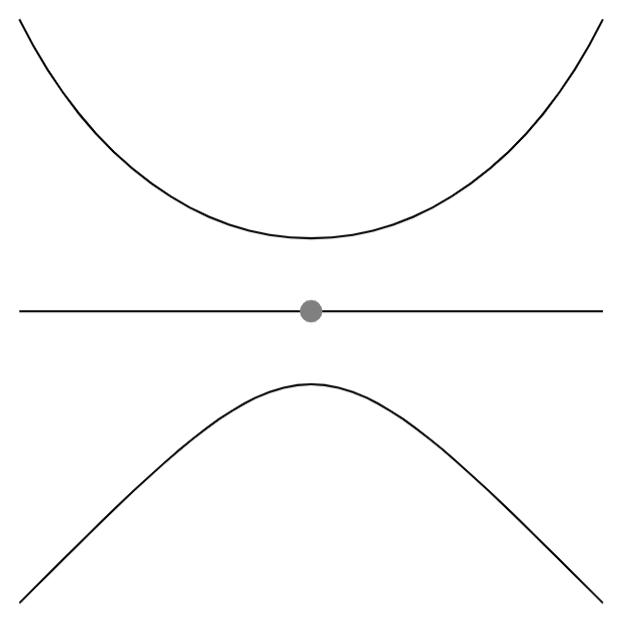There are three basic commands in this example:

• \draw (-2,0) -- (2,0);: This defines a line whose endpoint are (-2,0) and (2,0).
• \filldraw [gray] (0,0) circle (2pt);: The point is created as a very small gray circle centred at (0,0) and whose radius is (2pt). The \filldraw command is used to draw elements and fill them with a specific colour. See the next section for more examples.
• \draw (-2,2) .. controls (-1,0) and (1,0) .. (2,2);: Draws a Bézier curve. There are 4 points defining it: (-2,2) and (2,2) are its endpoints, (-1,0) and (1,0) are control points that determine "how curved" it is. You can think of these two points as "attractor points".

## Basic geometric shapes: Circles, ellipses and polygons

Geometric figures can be constructed from simpler elements so let's start with circles, ellipses and arcs.

\documentclass{article}
\usepackage{tikz}
\begin{document}
\begin{tikzpicture}
\filldraw[color=red!60, fill=red!5, very thick](-1,0) circle (1.5);
\fill[blue!50] (2.5,0) ellipse (1.5 and 0.5);
\draw[ultra thick, ->] (6.5,0) arc (0:220:1);
\end{tikzpicture}
\end{document}


This example produces the following output: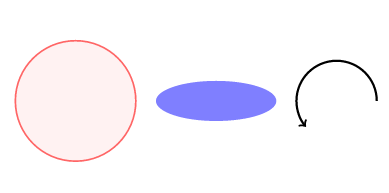• \filldraw[color=red!60, fill=red!5, very thick](-1,0) circle (1.5);: This command was used in the previous section to draw a point, but in this instance there are some additional parameters inside the brackets. These are explained below:
• color=red!60: The colour of the ring around the circle is set to 60% red (lighter than "pure" red). See the reference guide for a list of the default colours available in LaTeX; also, see Using colours in LaTeX to learn how to create your own colours.
• fill=red!5: The circle is filled with an even lighter shade of red.
• very thick: This parameter defines the thickness of the stroke. See the reference guide for a complete list of values.
• \fill[blue!50] (2.5,0) ellipse (1.5 and 0.5);: To create an ellipse you provide a centre point (2.5,0), and two radii: horizontal and vertical (1.5 and 0.5 respectively). Also notice the command fill instead of draw or filldraw, this is because, in this case, there's no need to control outer and inner colours.
• \draw[ultra thick, ->] (6.5,0) arc (0:220:1);: This command will draw an arc starting at (6.5,0). The extra parameter  ->  indicates that the arc will have an arrow at the end. In addition to the starting point you must provide three additional values: the starting and ending angles, and the radius; here, these three parameter values are provided in the format (0:220:1).

In addition to curved geometric shapes you can also create elements that use straight lines, using a similar syntax:

\documentclass{article}
\usepackage{tikz}
\begin{document}
\begin{tikzpicture}
\draw[blue, very thick] (0,0) rectangle (3,2);
\draw[orange, ultra thick] (4,0) -- (6,0) -- (5.7,2) -- cycle;
\end{tikzpicture}
\end{document}


This example produces the following output: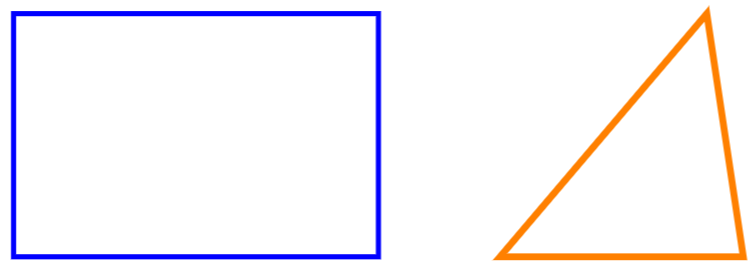• \draw[blue, very thick] (0,0) rectangle (3,2);: Rectangles are created by the special command rectangle. You have to provide two points, the first one is where the "pencil" begins to draw the rectangle and the second one is the diagonally opposite corner point.
• \draw[orange, ultra thick] (4,0) -- (6,0) -- (5.7,2) -- cycle;: To draw a polygon we draw a closed path of straight lines: a line from (4,0) to (6,0) and a line from (6,0) to (5.7,2). The cycle instruction means that the start and end points should coincide to create a "closed" path (shape), which results in construction of the final line segment.

## Diagrams

Nodes are probably the most versatile elements in TikZ. We've already used one node in the introduction—to add some text to the figure. The next example uses nodes to create a diagram.

\documentclass{article}
\usepackage{tikz}
\usetikzlibrary{positioning}
\begin{document}
\begin{tikzpicture}[
roundnode/.style={circle, draw=green!60, fill=green!5, very thick, minimum size=7mm},
squarednode/.style={rectangle, draw=red!60, fill=red!5, very thick, minimum size=5mm},
]
%Nodes
\node[squarednode]      (maintopic)                              {2};
\node[roundnode]        (uppercircle)       [above=of maintopic] {1};
\node[squarednode]      (rightsquare)       [right=of maintopic] {3};
\node[roundnode]        (lowercircle)       [below=of maintopic] {4};

%Lines
\draw[->] (uppercircle.south) -- (maintopic.north);
\draw[->] (maintopic.east) -- (rightsquare.west);
\draw[->] (rightsquare.south) .. controls +(down:7mm) and +(right:7mm) .. (lowercircle.east);
\end{tikzpicture}
\end{document}


This example produces the following output: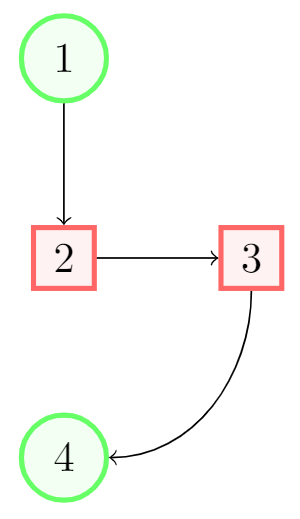There are essentially three commands in this figure: A node definition, a node declaration and lines that join two nodes.

• roundnode/.style={circle, draw=green!60, fill=green!5, very thick, minimum size=7mm}: Passed as a parameter to the tikzpicture environment. It defines a node that will be referenced as roundnode: this node will be a circle whose outer ring will be drawn using the colour green!60 and will be filled using green!5. The stroke will be very thick and its minimum size is 7mm. The line below this defines a second rectangle-shaped node called squarednode, using similar parameters.
• \node[squarednode] (maintopic) {2};: This will create a squarednode, as defined in the previous command. This node will have an id of maintopic and will contain the number 2. If you leave an empty space inside the braces no text will be displayed.
• [above=of maintopic]: Notice that all but the first node have an additional parameter that determines its position relative to other nodes. For instance, [above=of maintopic] means that this node should appear above the node named maintopic. For this positioning system to work you have to add \usetikzlibrary{positioning} to your preamble. Without the positioning library, you can use the syntax above of=maintopic instead, but the positioning syntax is more flexible and powerful: you can extend it to write above=3cm of maintopic i.e. control the actual distance from maintopic.
• \draw[->] (uppercircle.south) -- (maintopic.north);: An arrow-like straight line will be drawn. The syntax has been already explained in the basic elements section. The only difference is the manner in which we write the endpoints of the line: by referencing a node (this is why we named them) and a position relative to the node.

## Reference Guide

Possible color and thickness parameters in the tikz package:

parameter values picture
color white, black, red, green, blue, cyan, magenta, yellow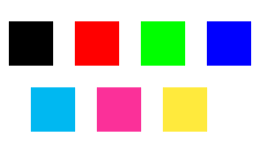thickness ultra thin, very thin, thin, thick, very thick, ultra thick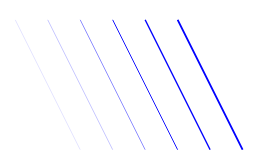More colours may be available in your LaTeX distribution. See Using colours in LaTeX